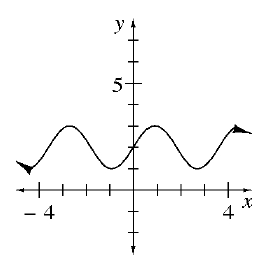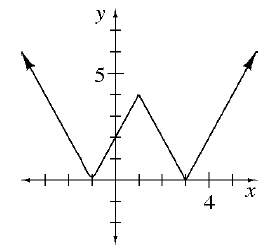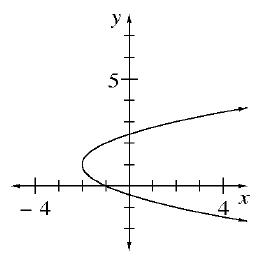### Home > GB8I > Chapter 1 Unit 1 > Lesson INT1: 1.3.2 > Problem1-83

1-83.

1.1.1.• Do all of these graphs have one output value for every input value?

1. For each graph above, state the domain and range.

• The domain of a graph is its possible $x$-values.

All real numbers.

The range of a graph is its possible y-values.

$1\le y\le3$